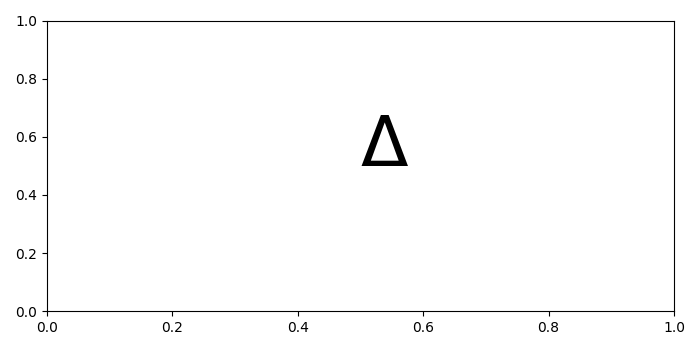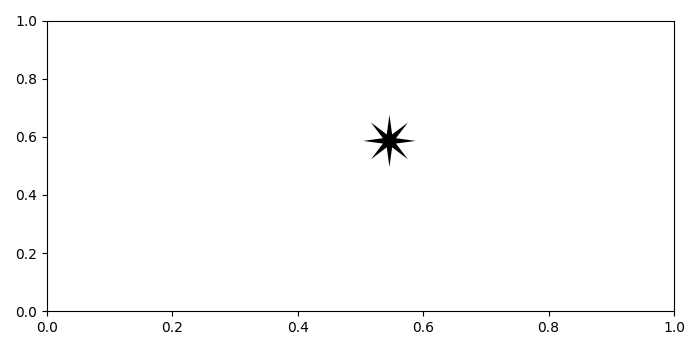# How to use unicode symbols in matplotlib?

To use unicode symbols in matplotlib, we can take the following steps.

## Steps

• Set the figure size and adjust the padding between and around the subplots.

• Add text to figure, using text() method with unicode symbols. Here we have used the Unicode chararacter (Δ) which has the character code (0394).

• To display the figure, use Show() method.

## Example

import matplotlib.pyplot as plt

# Set the figure size
plt.rcParams["figure.figsize"] = [7.00, 3.50]
plt.rcParams["figure.autolayout"] = True

# Unicode symbol
plt.text(0.5, 0.5, s=u"\u0394", fontsize=50)

# Display the plot
plt.show()


## Output

It will produce the following output −Now, let's use another Unicode character (\u2734).

It will produce the following output −